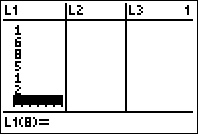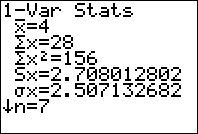﻿ Standard Deviation and Variance on a Graphing Calulator

# Find the Standard Deviation With a Graphing Calculator (TI83 or TI84)

Only the truly insane (or those in an introductory statistics course) would calculate the standard deviation of a dataset by hand! So what is left for the rest of us level headed folks? Statisticians typically use software like R or SAS, but in a classroom there isn’t always access to a full PC. Instead, we can use a graphing calculator to perform the exact same calculations. Note: You can scroll down for a video walkthrough of these steps.

## Standard Deviation on the TI83 or TI84

For this example, we will use a simple made-up data set: 5, 1, 6, 8, 5, 1, 2. For now, we won’t concern ourselves with whether this is sample or population data. This will come up later in the steps.

### Step 1: Enter your data into the calculator.

This will be the first step for any calculations on data using your calculator. To get to the menu to enter data, press [STAT] and then select 1:Edit.Now, we can type in each number into the list L1. After each number, hit the [ENTER] key to go to the next line. The entire dataset should go into L1. If for some reason, you don’t see L1, see: Getting L1 on Your Calculator.### Step 2: Calculate 1-Variable Statistics

Once the data is entered, hit [STAT] and then go to the CALC menu (at the top of the screen). Finally, select 1-var-stats and then press [ENTER] twice.### Step 3: Select the correct standard deviation

Now we have to be very careful. There are two standard deviations listed on the calculator. The symbol Sx stands for sample standard deviation and the symbol σ stands for population standard deviation. If we assume this was sample data, then our final answer would be s =2.71. Pay attention to what kind of data you are working with and make sure you select the correct one! In some cases, you are working with population data and will select σ.

## What about the variance?

The variance does not come out on this output, however it can always be found using one important property:

$$\text{Variance} = \text{(Standard Deviation)}^2$$

So in this example, the variance is:

$$s^2 = 2.71^2 = 7.34$$

This would work even if it was population data, but the symbol would be $$\sigma^2$$.

## Video walkthrough

The following video will walk your through all of these steps. Make sure you have your calculator ready to follow along!## Subscribe to our Newsletter!

We are always posting new free lessons and adding more study guides, calculator guides, and problem packs.

Sign up to get occasional emails (once every couple or three weeks) letting you know what's new!